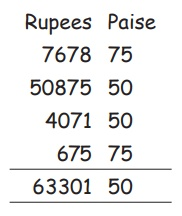Home | | Maths 5th Std | Addition and Subtraction using money

# Addition and Subtraction using money

We have already learnt about the conversion of money in the interconcept chapter in the previous term.

UNIT−5

MONEYRecall:

We have already learnt about the conversion of money in the interconcept chapter in the previous term. Let us try to fill in the following.

Try this

1 = 100 Paise

5 = 500 Paise

775 Paise = 7.75

425 Paise = 4.25Situation 1

Sarathi and Meenu went to a temple car festival (Thaer thiruvizha) with their parents. In the festival, Sarathi bought a watch for  70, Meenu bought bangles and earrings for  90 and their mother bought a chain for 160. The shopkeeper asked them to pay money for what they bought. Meenu didn’t know how much money she had to pay. So, she asked her mother. Her mother told her to add the cost of the items purchased. She added as follows.

Cost of the watch =  70

Cost of bangles and earrings = 90

Cost of the chain = 160

Total cost = 70 + 90 + 160 = 320.

So Meenu had to pay 320 to the shopkeeper.

Situation 2

Vasu had a stationery shop. He wanted to check the stock of pen. Vasu didn’t know how to find out the remaining items. For that he asked his friend. His friend helped him to form a table. The table is given belowTo get the cost of remaining item, we have to subtract the cost of sold out items from the cost of available items.

That is, cost of remaining items = cost of available item – cost of sold out items

= 3750 – 1680

= 2070

Example 5.1

Arun bought a T.V, a Washing Machine and a bike at the cost of 12,500, 14,999 and 75,000 respectively. Find the total amount spent by Arun.

Solution

Cost of a T.V                       = ₹ 12,500

Cost of a washing mechine = ₹ 14,999(+)

Cost of a Bike                        = ₹ 75,000(+)

Total amount                          = ₹ 1,02,499

Example 5.2

Find the total amount by adding the amounts 7678.75, 50875.50, 4071.50 and 675.75

SolutionAdd this paise 75+50+50+75 and covert into rupees.

75+50+50+75 = 250 paise

100 paise = 1 rupees

250 paise = 250/100 = 2.50 rupees

Therefore, the total amout = ₹ 7678.75 + ₹50875.50 + ₹407.50+ ₹675.75 = ₹63,301.50

Example 5.3

A colony of people had arranged for a tour for three days. They allotted an amount of rupees  18,540 for the same. They spent  6235 on the first day. How much money was left for the next two days?

Solution

Amount allotted for three days = 18,540

Amount spent on the first day =   6,235

Remaining amount                    = 12,305

Therefore, remaining amount for the next two days is  12,305.

Example 5.4

Geetha wants to buy a table for her family. She has paid an amount of  4,529.50 to the Shop Keeper. He has given  439.75 as balance to her. What is the cost of the table?

Solution

Amount paid by Geetha                                = ₹4,529.50

Balance amount given by the shop keeper = ₹ 439.75 (−)

Cost of the table                                            = ₹ 4,089.75

When we subtract 75 paise from 50 paise convert ₹ 1 into paise and add with 50 paise and then subtract.

₹ 1 = 100 paise

100+50=150 paise

150−75 =75 paise

Activity

Match the big bag with small bagsTags : Money | Term 3 Chapter 5 | 5th Maths , 5th Maths : Term 3 Unit 5 : Money
Study Material, Lecturing Notes, Assignment, Reference, Wiki description explanation, brief detail
5th Maths : Term 3 Unit 5 : Money : Addition and Subtraction using money | Money | Term 3 Chapter 5 | 5th Maths